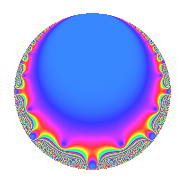# Properties

 Label 12.4.aLevel 12 Weight 4 Character orbit a Rep. character $$\chi_{12}(1,\cdot)$$ Character field $$\Q$$ Dimension 1 Newforms 1 Sturm bound 8 Trace bound 0

# Related objects

## Defining parameters

 Level: $$N$$ = $$12 = 2^{2} \cdot 3$$ Weight: $$k$$ = $$4$$ Character orbit: $$[\chi]$$ = 12.a (trivial) Character field: $$\Q$$ Newforms: $$1$$ Sturm bound: $$8$$ Trace bound: $$0$$

## Dimensions

The following table gives the dimensions of various subspaces of $$M_{4}(\Gamma_0(12))$$.

Total New Old
Modular forms 9 1 8
Cusp forms 3 1 2
Eisenstein series 6 0 6

The following table gives the dimensions of the cuspidal new subspaces with specified eigenvalues for the Atkin-Lehner operators and the Fricke involution.

$$2$$$$3$$FrickeDim.
$$-$$$$-$$$$+$$$$1$$
Plus space$$+$$$$1$$
Minus space$$-$$$$0$$

## Trace form

 $$q$$ $$\mathstrut +\mathstrut 3q^{3}$$ $$\mathstrut -\mathstrut 18q^{5}$$ $$\mathstrut +\mathstrut 8q^{7}$$ $$\mathstrut +\mathstrut 9q^{9}$$ $$\mathstrut +\mathstrut O(q^{10})$$ $$q$$ $$\mathstrut +\mathstrut 3q^{3}$$ $$\mathstrut -\mathstrut 18q^{5}$$ $$\mathstrut +\mathstrut 8q^{7}$$ $$\mathstrut +\mathstrut 9q^{9}$$ $$\mathstrut +\mathstrut 36q^{11}$$ $$\mathstrut -\mathstrut 10q^{13}$$ $$\mathstrut -\mathstrut 54q^{15}$$ $$\mathstrut +\mathstrut 18q^{17}$$ $$\mathstrut -\mathstrut 100q^{19}$$ $$\mathstrut +\mathstrut 24q^{21}$$ $$\mathstrut +\mathstrut 72q^{23}$$ $$\mathstrut +\mathstrut 199q^{25}$$ $$\mathstrut +\mathstrut 27q^{27}$$ $$\mathstrut -\mathstrut 234q^{29}$$ $$\mathstrut -\mathstrut 16q^{31}$$ $$\mathstrut +\mathstrut 108q^{33}$$ $$\mathstrut -\mathstrut 144q^{35}$$ $$\mathstrut -\mathstrut 226q^{37}$$ $$\mathstrut -\mathstrut 30q^{39}$$ $$\mathstrut +\mathstrut 90q^{41}$$ $$\mathstrut +\mathstrut 452q^{43}$$ $$\mathstrut -\mathstrut 162q^{45}$$ $$\mathstrut +\mathstrut 432q^{47}$$ $$\mathstrut -\mathstrut 279q^{49}$$ $$\mathstrut +\mathstrut 54q^{51}$$ $$\mathstrut +\mathstrut 414q^{53}$$ $$\mathstrut -\mathstrut 648q^{55}$$ $$\mathstrut -\mathstrut 300q^{57}$$ $$\mathstrut -\mathstrut 684q^{59}$$ $$\mathstrut +\mathstrut 422q^{61}$$ $$\mathstrut +\mathstrut 72q^{63}$$ $$\mathstrut +\mathstrut 180q^{65}$$ $$\mathstrut +\mathstrut 332q^{67}$$ $$\mathstrut +\mathstrut 216q^{69}$$ $$\mathstrut -\mathstrut 360q^{71}$$ $$\mathstrut +\mathstrut 26q^{73}$$ $$\mathstrut +\mathstrut 597q^{75}$$ $$\mathstrut +\mathstrut 288q^{77}$$ $$\mathstrut +\mathstrut 512q^{79}$$ $$\mathstrut +\mathstrut 81q^{81}$$ $$\mathstrut -\mathstrut 1188q^{83}$$ $$\mathstrut -\mathstrut 324q^{85}$$ $$\mathstrut -\mathstrut 702q^{87}$$ $$\mathstrut -\mathstrut 630q^{89}$$ $$\mathstrut -\mathstrut 80q^{91}$$ $$\mathstrut -\mathstrut 48q^{93}$$ $$\mathstrut +\mathstrut 1800q^{95}$$ $$\mathstrut -\mathstrut 1054q^{97}$$ $$\mathstrut +\mathstrut 324q^{99}$$ $$\mathstrut +\mathstrut O(q^{100})$$

## Decomposition of $$S_{4}^{\mathrm{new}}(\Gamma_0(12))$$ into irreducible Hecke orbits

Label Dim. $$A$$ Field CM Traces A-L signs $q$-expansion
$$a_2$$ $$a_3$$ $$a_5$$ $$a_7$$ 2 3
12.4.a.a $$1$$ $$0.708$$ $$\Q$$ None $$0$$ $$3$$ $$-18$$ $$8$$ $$-$$ $$-$$ $$q+3q^{3}-18q^{5}+8q^{7}+9q^{9}+6^{2}q^{11}+\cdots$$

## Decomposition of $$S_{4}^{\mathrm{old}}(\Gamma_0(12))$$ into lower level spaces

$$S_{4}^{\mathrm{old}}(\Gamma_0(12)) \cong$$ $$S_{4}^{\mathrm{new}}(\Gamma_0(6))$$$$^{\oplus 2}$$